Matplotlib 设置坐标轴

5 篇文章 0 订阅

调整名字和间隔

import matplotlib.pyplot as plt
import numpy as np

x = np.linspace(-3, 3, 50)
y1 = 2*x + 1
y2 = x**2

plt.figure()
plt.plot(x, y2)
plt.plot(x, y1, color='red', linewidth=1.0, linestyle='--')

plt.xlim((-1, 2))
plt.ylim((-2, 3))
plt.xlabel('I am x')
plt.ylabel('I am y')
plt.show()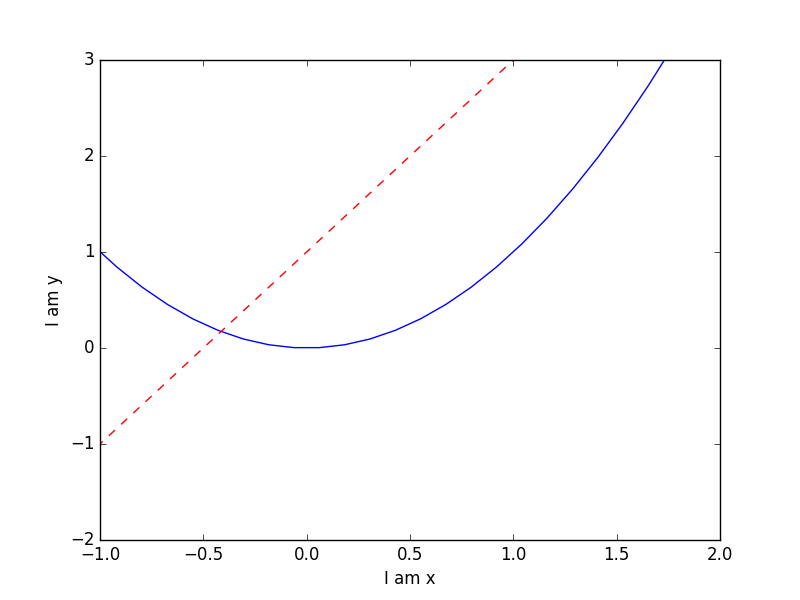new_ticks = np.linspace(-1, 2, 5)
print(new_ticks)
plt.xticks(new_ticks)

plt.yticks([-2, -1.8, -1, 1.22, 3],[r'$really\ bad$', r'$bad$', r'$normal$', r'$good$', r'$really\ good$'])
plt.show()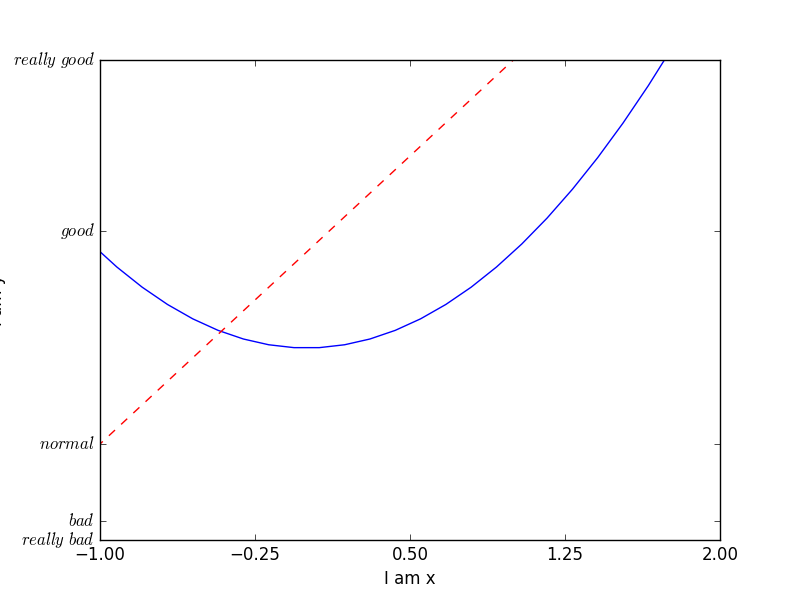设置不同名字和位置

import matplotlib.pyplot as plt
import numpy as np

x = np.linspace(-3, 3, 50)
y1 = 2*x + 1
y2 = x**2

plt.figure()
plt.plot(x, y2)
plt.plot(x, y1, color='red', linewidth=1.0, linestyle='--')
plt.xlim((-1, 2))
plt.ylim((-2, 3))

new_ticks = np.linspace(-1, 2, 5)
plt.xticks(new_ticks)
plt.yticks([-2, -1.8, -1, 1.22, 3],['$really\ bad$', '$bad$', '$normal$', '$good$', '$really\ good$'])

ax = plt.gca()
ax.spines['right'].set_color('none')
ax.spines['top'].set_color('none')
plt.show()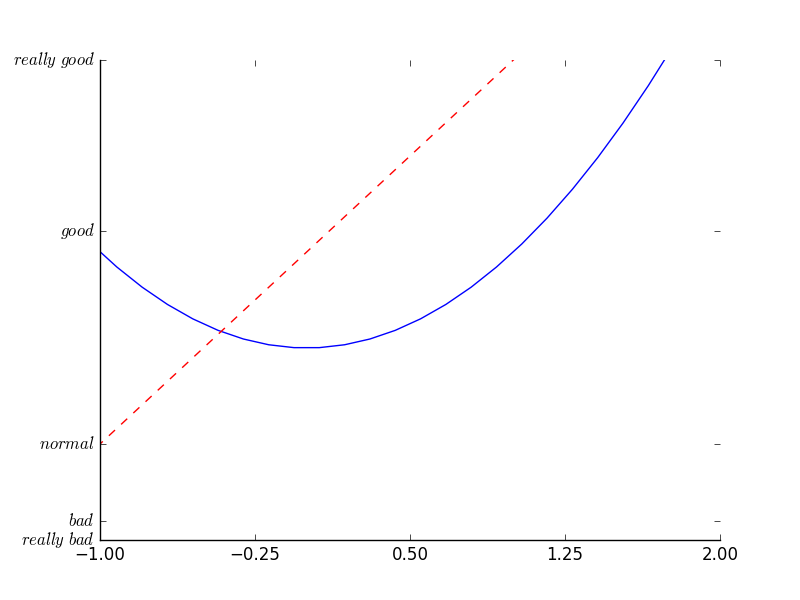调整坐标轴

ax.xaxis.set_ticks_position('bottom')

ax.spines['bottom'].set_position(('data', 0))
plt.show()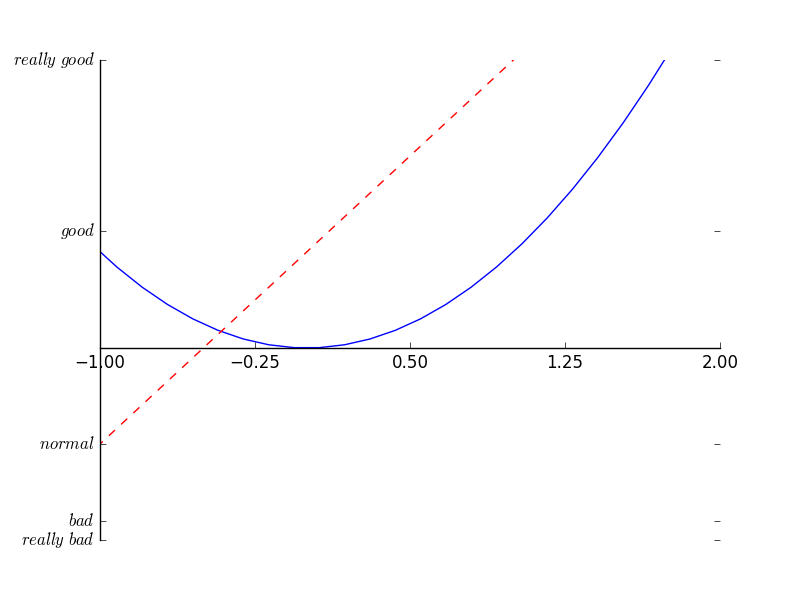ax.yaxis.set_ticks_position('left')

ax.spines['left'].set_position(('data',0))
plt.show()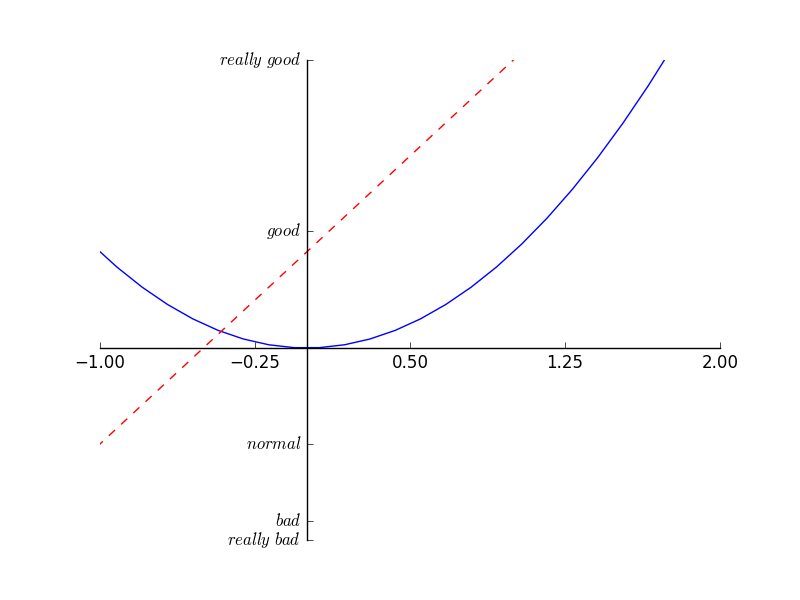05-0415万+10-121万+
03-277978
03-203806
11-291万+
10-159214
12-0374
05-071万+
11-161万+
08-1315万+
02-249389
11-20394
07-161万+
09-022578
10-265707
12-10699
03-043066
02-186万+
©️2021 CSDN 皮肤主题: Age of Ai 设计师:meimeiellie点击重新获取扫码支付1.余额是钱包充值的虚拟货币，按照1:1的比例进行支付金额的抵扣。
2.余额无法直接购买下载，可以购买VIP、C币套餐、付费专栏及课程。余额充值